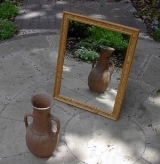xMirror imageOverview

A mirror image is a reflected duplication of an object that appears identical but reversed. As an optical
Optics
Optics is the branch of physics which involves the behavior and properties of light, including its interactions with matter and the construction of instruments that use or detect it. Optics usually describes the behavior of visible, ultraviolet, and infrared light...

effect it results from reflection
Reflection (physics)
Reflection is the change in direction of a wavefront at an interface between two differentmedia so that the wavefront returns into the medium from which it originated. Common examples include the reflection of light, sound and water waves...

off of substances such as a mirror
Mirror
A mirror is an object that reflects light or sound in a way that preserves much of its original quality prior to its contact with the mirror. Some mirrors also filter out some wavelengths, while preserving other wavelengths in the reflection...

or water. It is also a concept in geometry
Geometry
Geometry arose as the field of knowledge dealing with spatial relationships. Geometry was one of the two fields of pre-modern mathematics, the other being the study of numbers ....

and can be used as a conceptualization process for 3-D structures. In chemistry
Chemistry
Chemistry is the science of matter, especially its chemical reactions, but also its composition, structure and properties. Chemistry is concerned with atoms and their interactions with other atoms, and particularly with the properties of chemical bonds....

, a '"mirror image'" is a molecule having a spatial arrangement that corresponds to that of another molecule except that the right-to-left sense on one corresponds to the left-to-right sense on the other, the left handedness being known as "levo" or l-x and the righthandedness being known as "dextro" or d-x, where "x" is the referenced molecule.
In geometry
Geometry
Geometry arose as the field of knowledge dealing with spatial relationships. Geometry was one of the two fields of pre-modern mathematics, the other being the study of numbers ....

, the mirror image of an object or two-dimensional figure
2D geometric model
A 2D geometric model is a geometric model of an object as two-dimensional figure, usually on the Euclidean or Cartesian plane.Even though all material objects are three-dimensional, a 2D geometric model is often adequate for certain flat objects, such as paper cut-outs and machine parts made of...

is the virtual image
Virtual image
In optics, a virtual image is an image in which the outgoing rays from a point on the object always diverge. It will appear to converge in or behind the optical device . A simple example is a flat mirror where the image of oneself is perceived at twice the distance from oneself to the mirror...

formed by reflection
Reflection (mathematics)
In mathematics, a reflection is a mapping from a Euclidean space to itself that is an isometry with a hyperplane as set of fixed points; this set is called the axis or plane of reflection. The image of a figure by a reflection is its mirror image in the axis or plane of reflection...

in a plane mirror
Plane mirror
A plane mirror is a mirror with a plane reflective surface.For light rays striking a plane mirror, the angle of reflection equals the angle of incidence...

; it is of the same size as the original object, yet different, unless the object or figure has reflection symmetry
Reflection symmetry
Reflection symmetry, reflectional symmetry, line symmetry, mirror symmetry, mirror-image symmetry, or bilateral symmetry is symmetry with respect to reflection. That is, a figure which does not change upon undergoing a reflection has reflectional symmetry.In 2D there is a line of symmetry, in 3D a...

(also known as a P-symmetry).

Two-dimensional mirror images can be seen in the reflections of mirrors or other reflecting surfaces, or on a printed surface seen inside out.
The concept of mirror image can be extended to three-dimensional
Three-dimensional space
Three-dimensional space is a geometric 3-parameters model of the physical universe in which we live. These three dimensions are commonly called length, width, and depth , although any three directions can be chosen, provided that they do not lie in the same plane.In physics and mathematics, a...

objects, including the inside parts, even if they are not transparent.